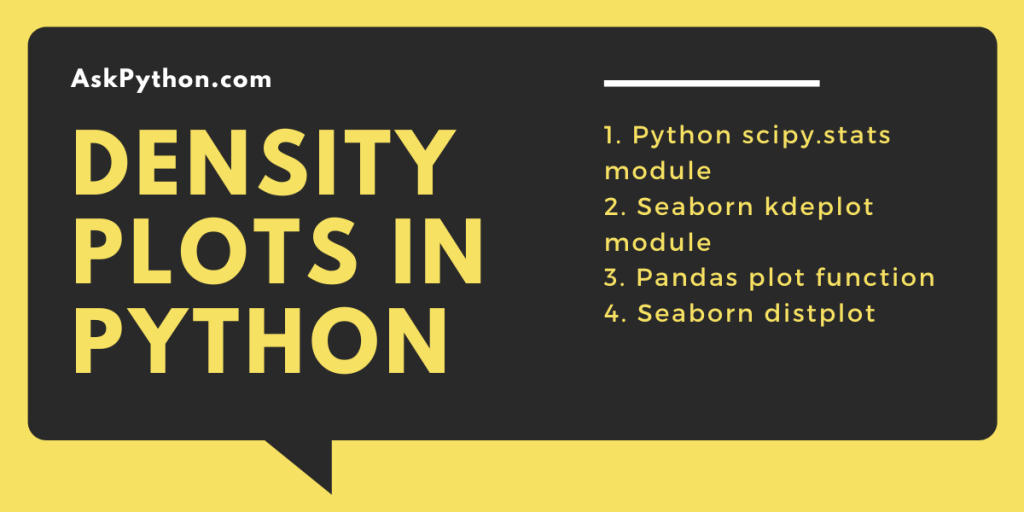# Density Plots in Python – A Comprehensive OverviewA density plot is used to visualize the distribution of a continuous numerical variable in a dataset. It is also known as Kernel Density Plots.

It’s a good practice to know your data well before starting to apply any machine learning techniques to it.

As a good ML practitioner we should be asking some questions like:

• What does our data look like?
• Is it normally distributed or have some different shape?
• The algorithms we are intending to apply to our data, does it has any underlying assumptions about the distribution of data?

Addressing such questions right after we acquire our data can drastically improve the results in later stages and save us a lot of time.

Plots like Histograms and Density plots serve us the ways to answer the questions mentioned above.

## Why understand histograms before learning about density plots?

A density plot is very analogous to a histogram. We visualize the shape of the distribution using a histogram. Histograms can be created by binning the data and keeping the count of the number of observations in each bin. In a histogram, the y-axis usually denotes bin counts, but can also be represented in counts per unit also called as densities.

If we increase the number of bins in our histogram, the shape of distribution appears to be smoother.

Now, imagine a smooth continuous line passing through top of each bin, creating an outline of the shape of our distribution. The result we get is what we call as a Density Plot.

## Understanding The Density Plot

We can think of density plots as plots of smoothened histograms, which is quite intuitive by now.  Density plots mostly use a kernel density estimate. Kernel density estimate allows smoother distributions by smoothing out the noise.

The density plots are not affected by the number of bins which is a major parameter when histograms are to be considered, hence allows us to better visualize the distribution of our data.

So in summary it is just like a histogram but having a smooth curve drawn through the top of each bin.

Several shapes of distributions exist out there in the wild. Some of the most common shapes that we would very likely to encounter are:

## Density Plots with Python

We can plot a density plot in many ways using python. Let’s look at a few commonly used methods.

### 1. Using Python scipy.stats module

`scipy.stats` module provides us with `gaussian_kde` class to find out density for a given data.

```import numpy as np
import matplotlib.pyplot as plt
from scipy.stats import gaussian_kde

data = np.random.normal(10,3,100) # Generate Data
density = gaussian_kde(data)

x_vals = np.linspace(0,20,200) # Specifying the limits of our data
density.covariance_factor = lambda : .5 #Smoothing parameter

density._compute_covariance()
plt.plot(x_vals,density(x_vals))
plt.show()

```

We change the function `covariance_factor` of the `gaussian_kde` class and pass on different values to get a smoother plot. Remember to call `_compute_covariance` after changing the function.

### 2. Using Seaborn `kdeplot` module

Seaborn module provides us with an easier way to execute the above task with much more flexibility.

```import numpy as np
import seaborn as sb
import matplotlib.pyplot as plt

data = np.random.normal(10,3,300) #Generating data.
plt.figure(figsize = (5,5))
sb.kdeplot(data , bw = 0.5 , fill = True)
plt.show()
```

Seaborn `kdeplot` requires a univariate data array or a pandas series object as an input argument to it. The `bw` argument is equivalent to `covariance_factor` of the `gaussian_kde` class demonstrated above. we can pass on `fill` = `False` to not fill the area under the curve with color and will simply plot a curve.

### 3. Using pandas plot function

Pandas `plot` method can also be used to plot density plots by providing `kind = 'density'` as an input argument to it.

```import numpy as np
import pandas as pd
import matplotlib.pyplot as plt

x_values = np.random.random(10,3,300) #Generating Data
df = pd.DataFrame(x_values, columns = ['var_name'] ) #Converting array to pandas DataFrame
df.plot(kind = 'density)
```

### 4. Using Seaborn `distplot`

We can also use the seaborn `distplot` method to visualize the distribution of continuous numerical data. `seaborn.distplot( )` method requires a univariate data variable as an input parameter which can be a pandas Series, 1d-array, or a list.

Some important arguments we can pass to `seaborn.distplot( )` to tweak the plot according to our needs are:

• `hist` : (Type – Bool) whether to plot a histogram or not.
• `kde` : (Type – Bool) whether to plot a gaussian kernel density estimate.
• `bins` : (Type – Number) specifying the number of bins in the histogram.
• `hist_kws` : (Type – Dict) dict of Keyword arguments for matplotlib.axes.Axes.hist()
• `kde_kws` : (Type – Dict) Keyword arguments for kdeplot() passed as a dictionary.
```import numpy as np
import matplotlib.pyplot as plt
import seaborn as sb

data = np.random.normal(10, 3, 1000) #Generating data randomly from a normal distribution.

sb.set_style("whitegrid")  # Setting style(Optional)
plt.figure(figsize = (10,5)) #Specify the size of figure we want(Optional)
sb.distplot(x = data  ,  bins = 10 , kde = True , color = 'teal'\
, kde_kws=dict(linewidth = 4 , color = 'black'))
plt.show()
```
To know more about seaborn `distplot` you can refer to this article on seaborn Distplots.